##### Economic Indicators For Dummies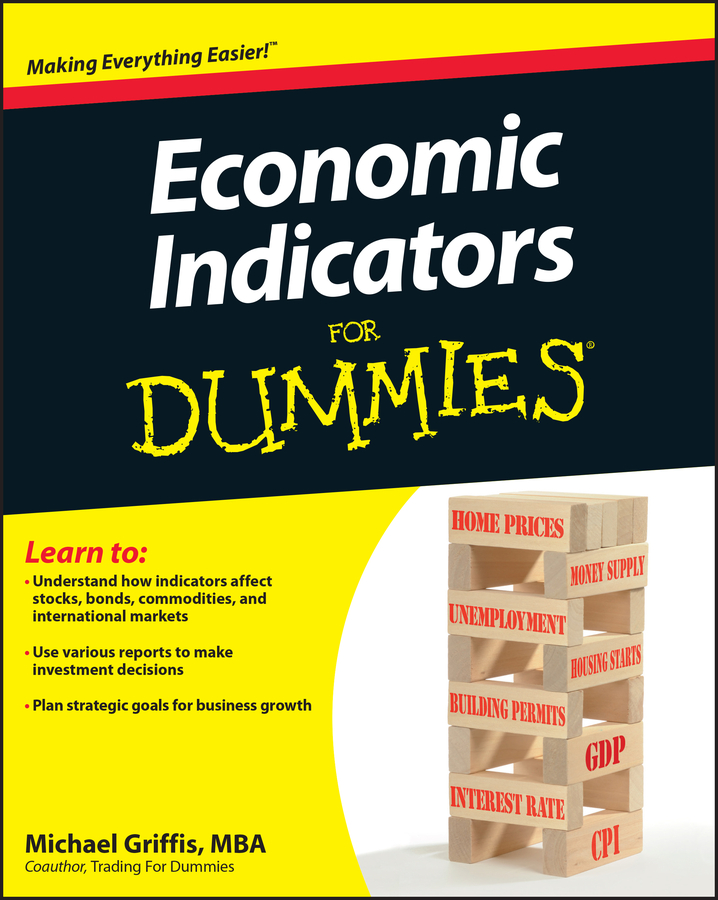Graphs easily describe the economic relationship between two variables; for example, a supply curve describes the relationship between price and quantity supplied. Economic relationships are also expressed as mathematical functions.

A function describes the relationship that exists between two or more variables. For example,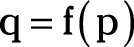is a general statement that indicates the variable q is a function of the variable p. A specific functional form describes the exact relationship that exists between those variables; the function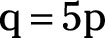indicates that for every one unit increase in p, q’s value increases by 5 units, or that q is five times greater than p. By identifying the exact relationship between variables, you’ve turned a general equation into a specific function.

Two variable functions are easily expressed with either an equation or a graph. However, for functional relationships involving three or more variables, you’ll find that equations are not only simpler than graphs, but also often a necessity. A functional relationship among three variables is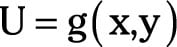This equation indicates that U is a function “g” of the variables x and y. In such functions, U is called the dependent variable because its value depends upon the values of x and y. The variables x and y are called independent variables because their values are given and determine U’s value.

Business decision-making requires knowing the specific relationship among variables. The equation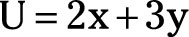indicates that a one-unit increase in the variable x causes a two-unit (2x) increase in U, while a one-unit increase in variable y causes a three-unit (3y) increase in U.